How to Calculate and Solve for the Water – Oil Ratio of a Reservoir Fluid Flow | The Calculator Encyclopedia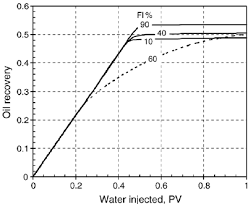The above image represents Water – Oil Ratio.

To compute for the water – oil ratio, six essential parameters are needed and these parameters are o)Oil Viscosity, (μw)Water Viscosity, (krw)Relative Permeability to Water, (kro)Relative Permeability to Oil, (Bw)Water Formation Volume Factor and (Bo)Oil Formation Volume Factor.

The formula for calculating the water – oil ratio:

WOR = [krw / kro] [μoBo  / μwBw ]

Where;

WOR = Water Oil Ratio
μo = Oil Viscosity
μw = Water Viscosity
krw = Relative Permeability to Water
kro = Relative Permeability to Oil
Bw = Water Formation Volume Factor
Bo = Oil Formation Volume Factor

Let’s solve an example;
Find the water – oil ratio when the oil viscosity is 9, water viscosity is 11 with a relative permeability to water of 21, relative permeability to oil of 7 and a water formation volume factor of 14 with oil formation volume factor of 27.

This implies that;

μo = Oil Viscosity = 9
μw = Water Viscosity = 11
krw = Relative Permeability to Water = 21
kro = Relative Permeability to Oil = 7
Bw = Water Formation Volume Factor = 14
Bo = Oil Formation Volume Factor = 27

WOR = [krw / kro] [μoBo  / μwBw ]
WOR = [21/7] [9 x 27 /11 x 14]
WOR = [21/7] [243/154]
WOR = [21/7] [1.5779]
WOR =  [1.5779]
WOR = 4.73

Therefore, the Water – Oil Ratio is 4.73.

Nickzom Calculator – The Calculator Encyclopedia is capable of calculating the water – oil ratio.

To get the answer and workings of the water – oil ratio using the Nickzom Calculator – The Calculator Encyclopedia. First, you need to obtain the app.

You can get this app via any of these means:

To get access to the professional version via web, you need to register and subscribe for NGN 1,500 per annum to have utter access to all functionalities.
You can also try the demo version via https://www.nickzom.org/calculator

Android (Paid) – https://play.google.com/store/apps/details?id=org.nickzom.nickzomcalculator
Android (Free) – https://play.google.com/store/apps/details?id=com.nickzom.nickzomcalculator
Apple (Paid) – https://itunes.apple.com/us/app/nickzom-calculator/id1331162702?mt=8
Once, you have obtained the calculator encyclopedia app, proceed to the Calculator Map, then click on Petroleum under EngineeringNow, Click on Reservoir Fluid Flow under Petroleum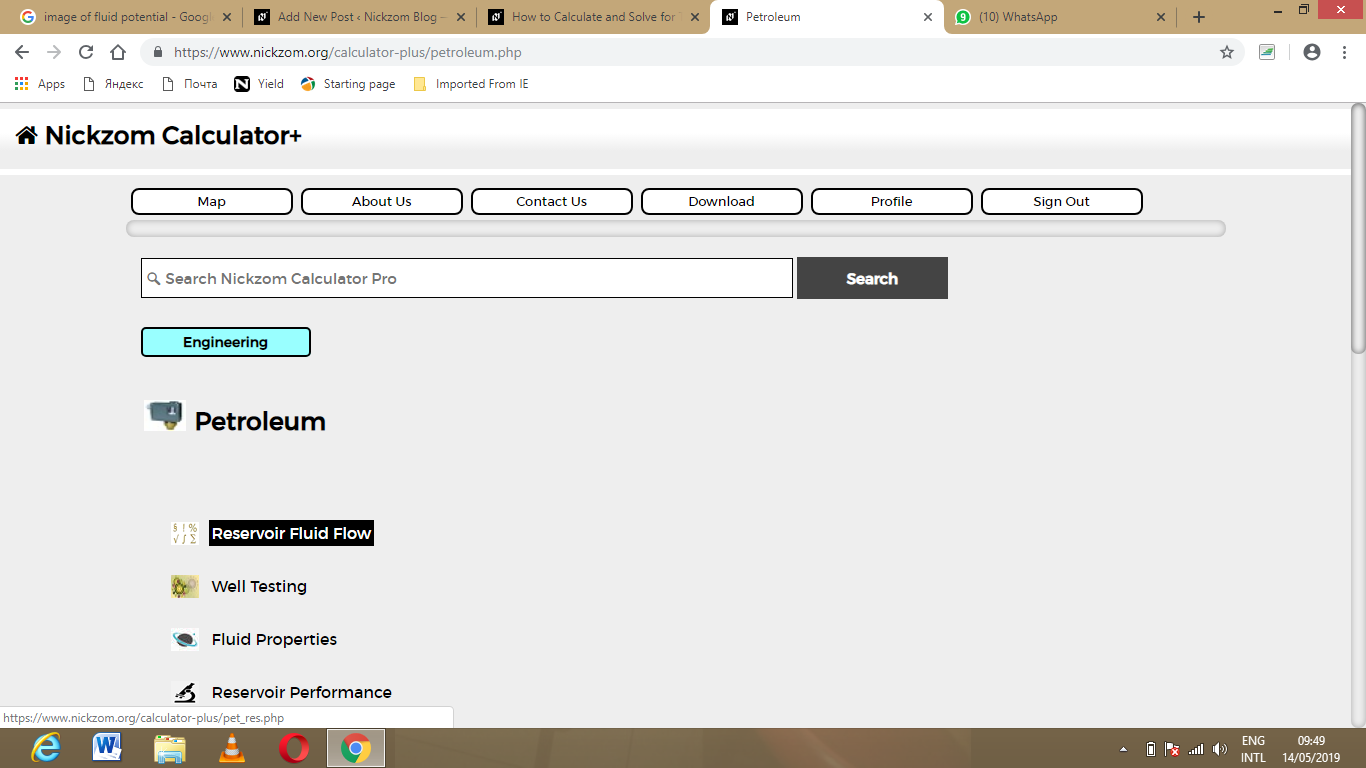Now, Click on Water – Oil Ratio under Reservoir Fluid Flow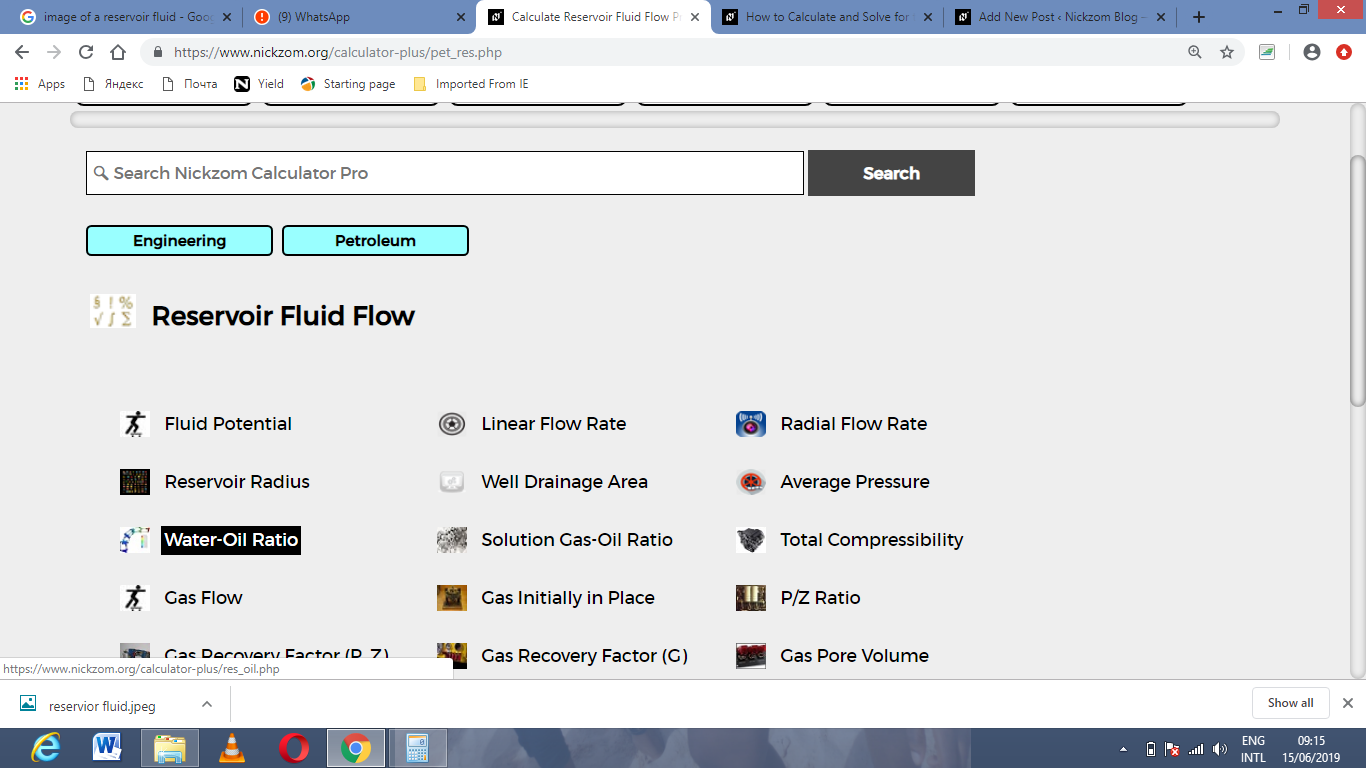The screenshot below displays the page or activity to enter your value, to get the answer for the water – oil ratio according to the respective parameter which are the o)Oil Viscosity, (μw)Water Viscosity, (krw)Relative Permeability to Water, (kro)Relative Permeability to Oil, (Bw)Water Formation Volume Factor and (Bo)Oil Formation Volume Factor.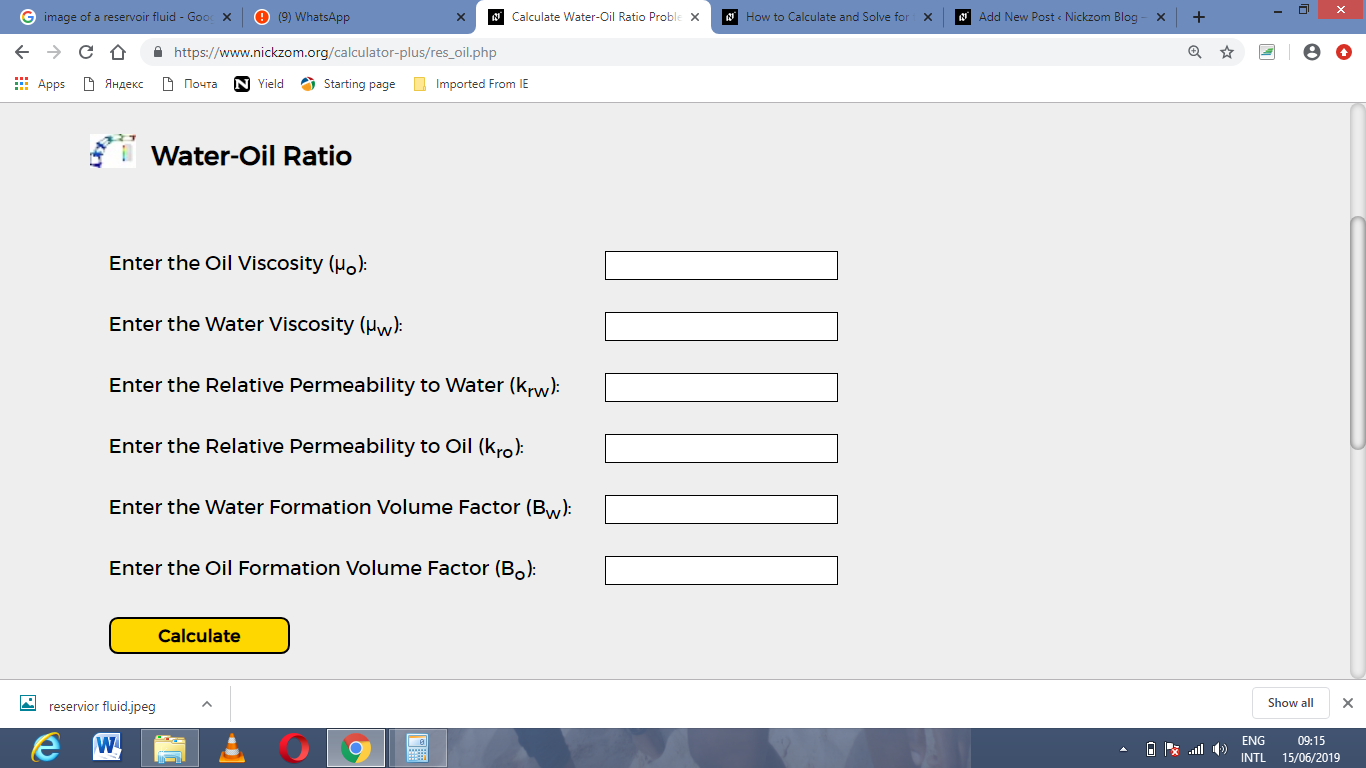Now, enter the value appropriately and accordingly for the parameter as required by the oil viscosity is 9, water viscosity is 11 with a relative permeability to water of 21, relative permeability to oil of 7 and a water formation volume factor of 14 with oil formation volume factor of 27.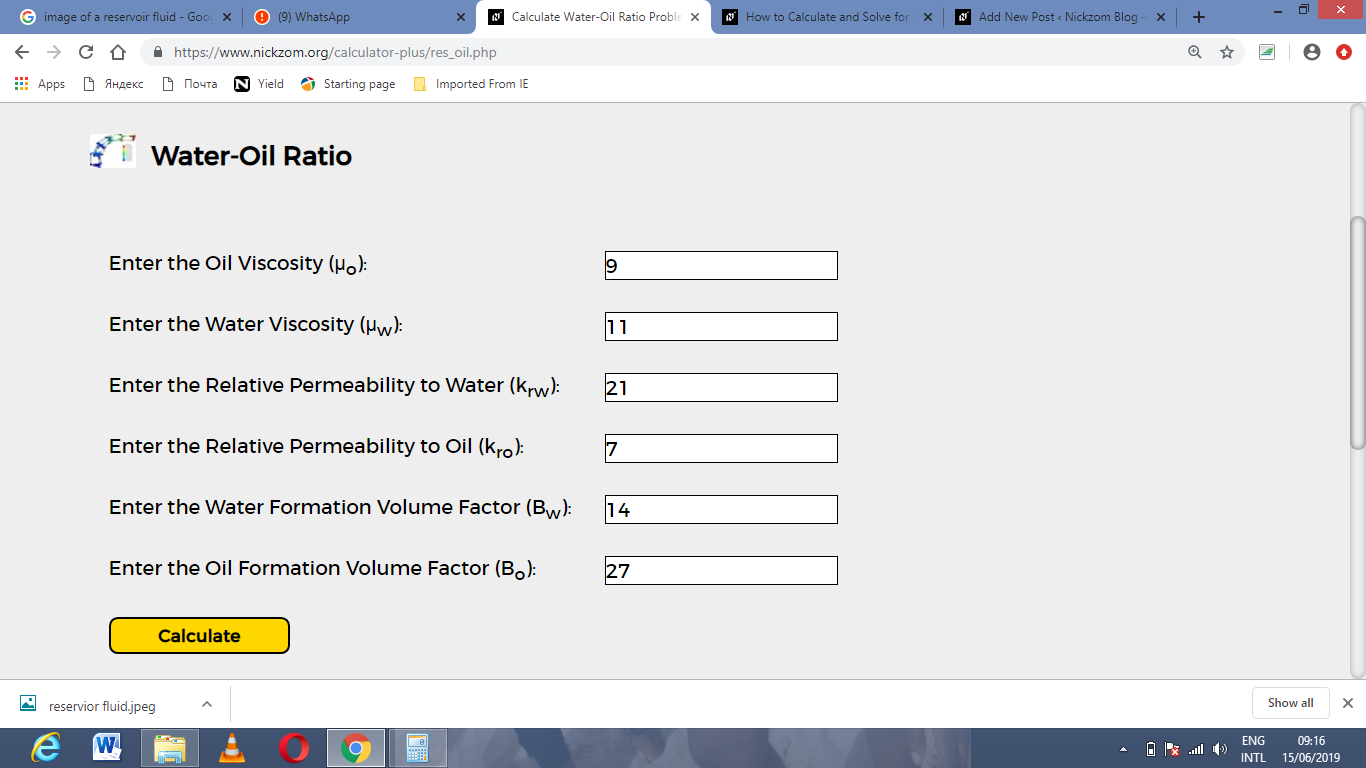Finally, Click on Calculate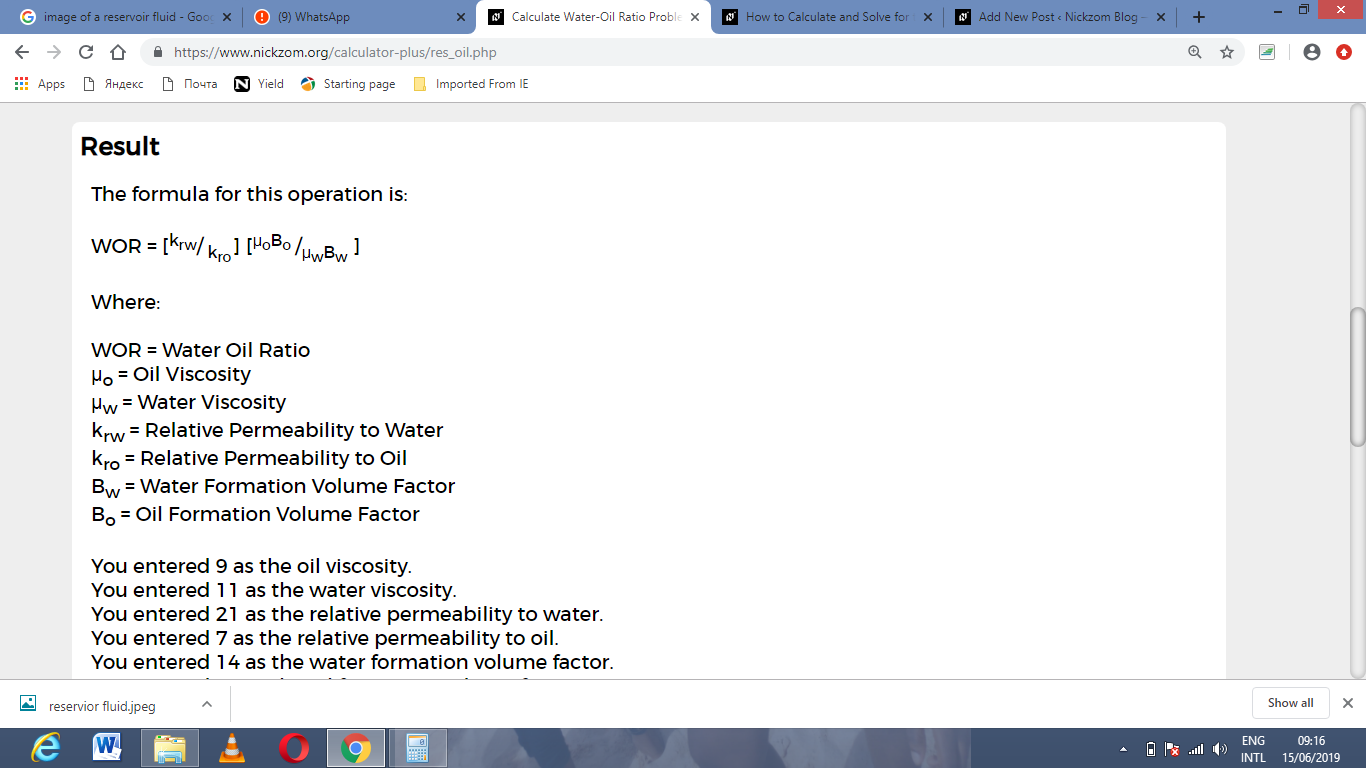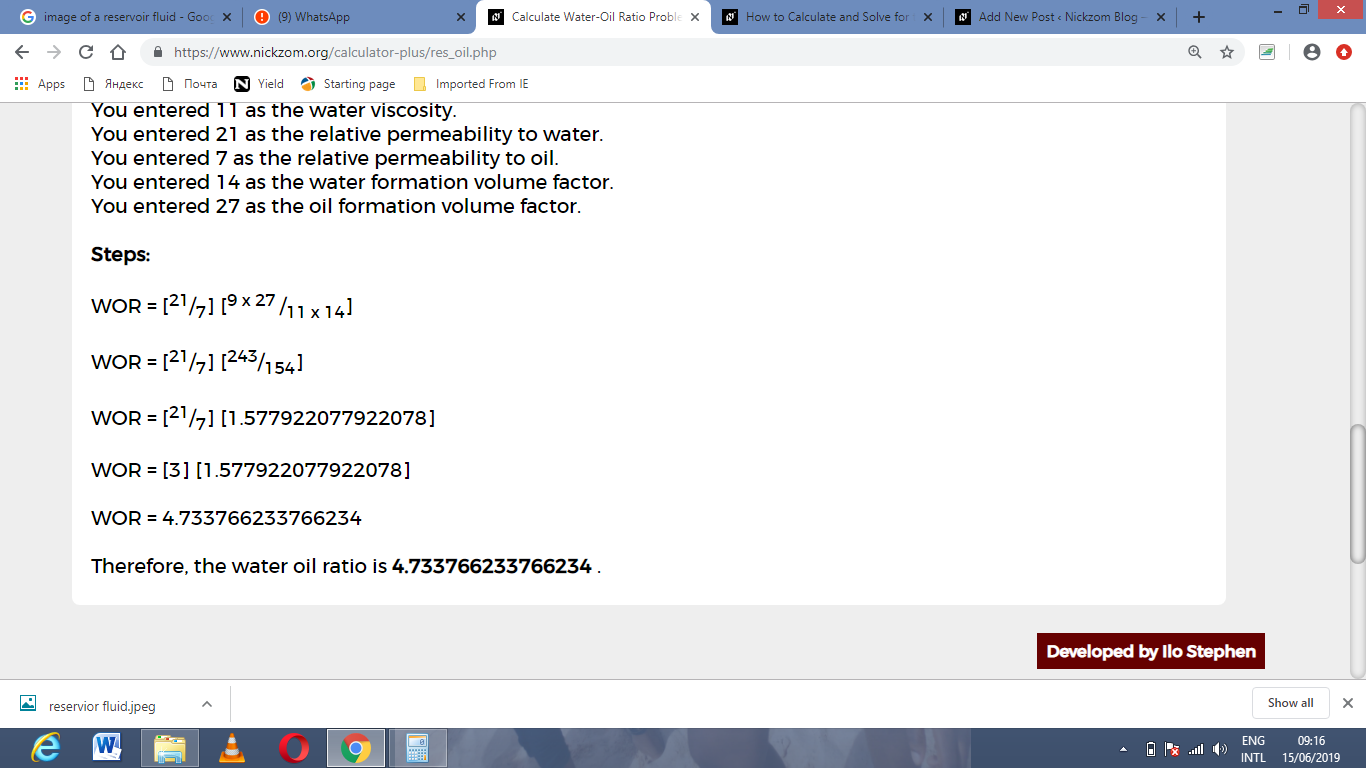As you can see from the screenshot above, Nickzom Calculator– The Calculator Encyclopedia solves for the water – oil ratio and presents the formula, workings and steps too.